GFG App
Open AppBrowser
Continue

A Triangle is a polygon with three sides. It is a 2-Dimensional polygon. Triangle is the simplest form of a Polygon. The word “Tri” means three and therefore a figure with 3 angles is a triangle, and It is formed with the help of three-line segments intersecting each other, a triangle has 3 vertices, 3 edges, and 3 angles.

The shape of a triangle is very useful in real life too, like carpentering, Astronomy, street signboards, etc.

## Triangle Definition

A triangle is a polygon with three sides and three angles. It is denoted by the symbol △.  In a triangle where any two-side joint is called a vertex. A triangle has three vertices. It is one of the basic figures used in mathematics, there are various properties of the triangle which are discussed below.

## Triangles Shape

Triangle shape is one of the most common shapes which we observe in our daily life. We observe traffic signals, snacks, cloth hangers, etc which are shaped like triangles. A triangle is a 2-Dimensional closed figure with three sides and three angles. It is the simplest polygon and is widely used in geometry and mathematics.

## Examples of Triangles

Various examples of triangles that we observed in our daily life include sandwiches, racks in billiards, tiles, and others. It is one of the fundamental shapes of nature and various shapes can be studied easily by dividing it into various triangles.

Some real-life examples of triangles are Nachos, Tiles, Photo frames, and others.

## Parts of a Triangle

A triangle as the name suggests has three angles thus it is called a “tri” angle. It contains various parts. A triangle has 3 angles, 3 vertices, and 3 sides.

In the triangle, ABC given in the image below shows the vertices, sides, and interior angles of the triangle.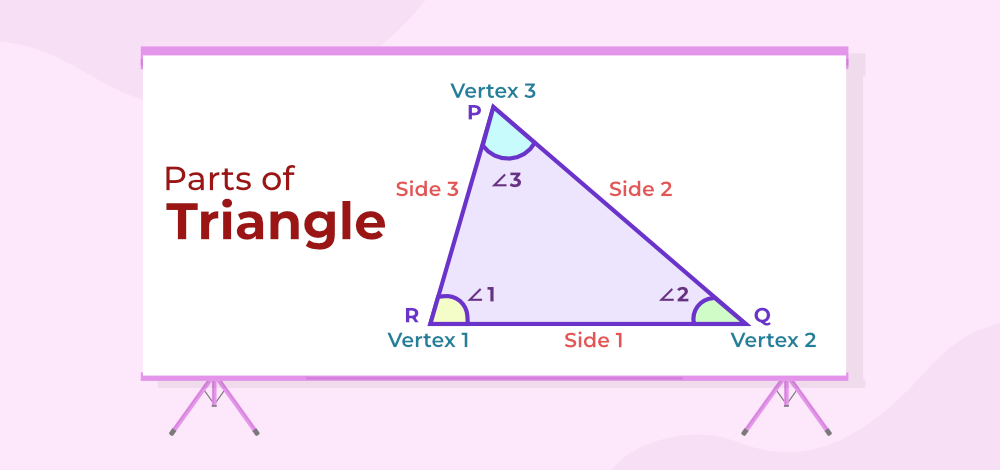• Three Angles of the triangle are, ∠ABC, ∠BCA, and ∠CAB.
• Three sides of the triangles are side AB, side BC, and side CA
• The three vertices of the triangle are A, B, and C.

## Angles in a Triangle

A triangle has three angles, an angle is formed when two sides of the triangle meet at a common point, this common point is known as the vertex. The sum of the three interior angles is equal to 180 degrees.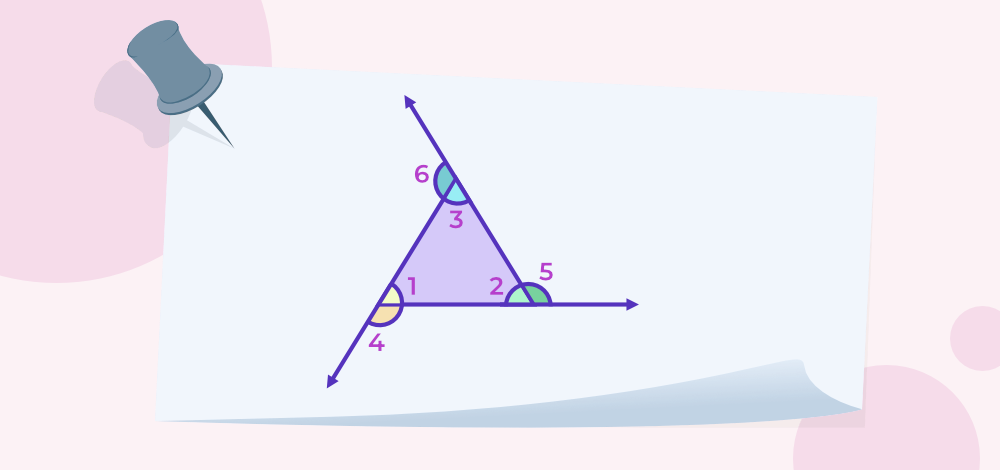Let’s take the triangle with the interior angles at ∠1, ∠2, and ∠3 and their respective exterior angle at, ∠4, ∠5 and ∠6

Now, using the triangle sum property

∠1 + ∠2 + ∠3 = 180°

When the sides are extended outwards in a triangle, then it forms three exterior angles. The sum of the interior and exterior angles pair of a triangle is always supplementary. Also, the sum of all three exterior angles of a triangle is 360 degrees.

Now,

∠4 + ∠5 + ∠6 = 360°

## Properties of a Triangle

• Angle Sum Property: The sum of all three interior angles is always 180°. Therefore. In the Triangle ΔABC shown above, ∠A+ ∠B+ ∠C= 180°, the interior angles of a triangle will be greater than 0° and less than 180°.
• A Triangle has 3 sides, 3 vertices, and 3 angles.
• Exterior angle property: The Exterior angle of a triangle is equal to the sum of Interior opposite and non-adjacent angles (also referred to as remote interior angles). In the above shown ΔABC, ∠ACD= ∠ABC+ ∠BAC
• The sum of the length of any two sides of a triangle is always greater than the third side. For example, AB+ BC> AC or BC+ AC> AB.
• The side opposite the largest angle is the largest side of the triangle. For instance, in a right-angled triangle, the side opposite 90° is the longest side.
• The perimeter of a figure is defined by the overall length the figure is covering. Hence, the perimeter of a triangle is equal to the sum of lengths on all three sides of the triangle. Perimeter of ΔABC= (AB + BC + AC)
• The difference between the length of any two sides is always lesser than the third side. For example, AB-BC< AC or BC-AC< AB
• For similar triangles, the angles of the two triangles have to be congruent to each other and the respective sides should be proportional.
• Area of Triangle: 1/2× base × height

## Triangle Formulas

In geometry, for every two-dimensional shape (2D shape), there are always two basic measurements that we need to find out, i.e., the area and perimeter of that shape. Therefore, the triangle has two basic formulas which help us to determine its area and perimeter. Let us discuss the formulas in detail.

### Perimeter of Triangle

Perimeter of a triangle is defined as the sum of all three sides of a triangle. Suppose a triangle with sides a, b, and c is given then its perimeter is given by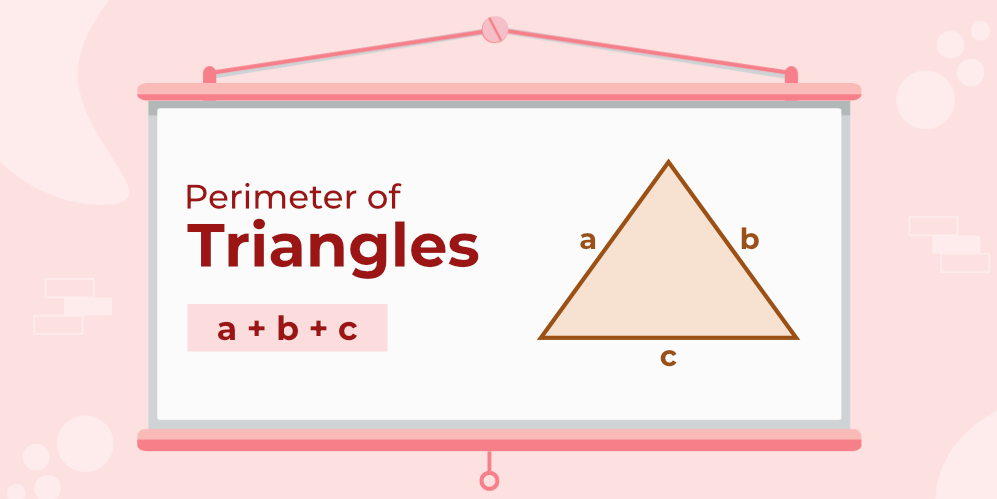Perimeter of triangle = a + b + c

### Area of a Triangle

Area of a triangle is the total area covered by the triangle boundary. It is equal to half the product of its base and height. Area of a triangle is measured in square units. If the base of a triangle is b and its height is h then its area is given by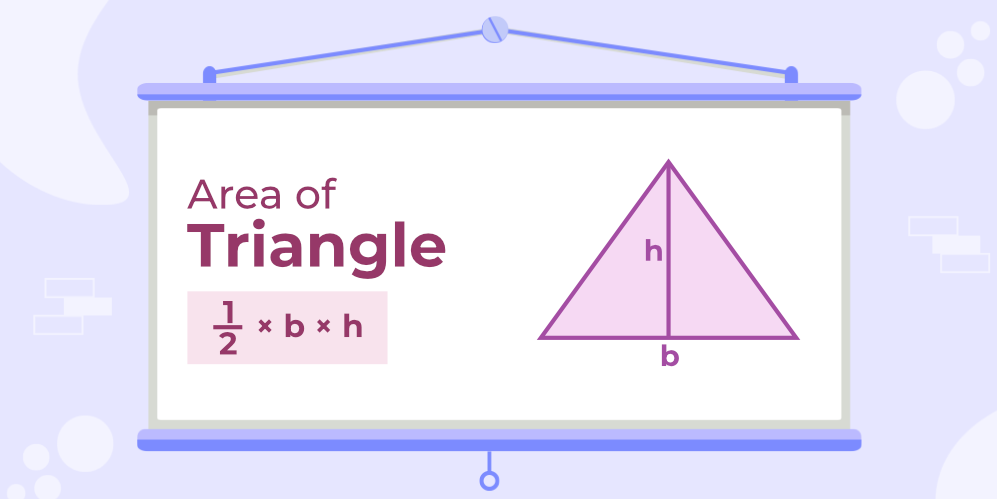Area of Δ = 1/2 × b × h

## Area of Triangle Using Heron’s Formula

When the three sides of a triangle are known but its height is unknown then its area is calculated using Heron’s Formula. Suppose a triangle with sides a, b, and c units is given then its area is calculated using the steps discussed below

### Steps to Find Area Using Herons Formula

Step 1: Mark all the dimensions of the given triangle.

Step 2: Calculate the semi-perimeter (s) using the formula s = (a+b+c) / 2

Step 3: Use the formula for finding area Area;

A = √[s(s-a)(s-b)(s-c)]

where,

a, b, and c are the sides of a triangle

## Types of Triangles

Classification of triangles is done based on the following characteristics:

• Types of Triangles Based on Sides
• Types of Triangles Based on Angles

## Types of Triangles Based on Sides

On the basis of sides, triangles are classified as

• Scalene Triangle
• Isosceles Triangle
• Equilateral Triangle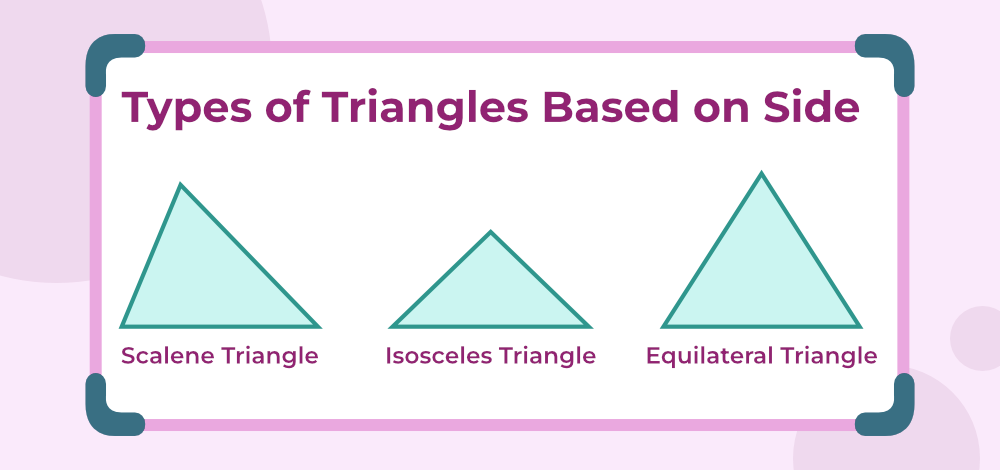### Equilateral Triangle

In an Equilateral triangle, all three sides are equal to each other as well as all three interior angles of the equilateral triangle are equal.

Since all the interior angles are equal and the sum of all the interior angles of a triangle is 180° (one of the Properties of the Triangle). We can calculate the individual angles of an equilateral triangle.

∠A+ ∠B+ ∠C = 180°

∠A = ∠B = ∠C

Therefore, 3∠A = 180°

∠A= 180/3 = 60°

Hence, ∠A = ∠B = ∠C = 60°

Properties of Equilateral Triangle

• All sides are equal.
• All angles are equal and are equal to 60°
• There exist three lines of symmetry in an equilateral triangle
• The angular bisector, altitude, median, and perpendicular line are all same and here it is AE.
• The orthocenter and centroid are the same.

Equilateral Triangle Formulas

The basic formulas for equilateral triangles are:

where,
a is the side of triangle

### Isosceles Triangle

In an Isosceles triangle, two sides are equal and the two angles opposite to the sides are also equal. It can be said that any two sides are always congruent. Area of an Isosceles triangle is calculated by using the formula for the area of the triangle as discussed above.

Properties of Isosceles Triangle

• Two sides of the isosceles triangle are always equal
• Third side is referred to as the base of the triangle and the height is calculated from the base to the opposite vertex
• Opposite angles corresponding to the two equal sides are also equal to each other.

### Scalene Triangle

In a Scalene triangle, all sides and all angles are unequal. Imagine drawing a triangle randomly and none of its sides is equal, all angles differ from each other too.

Properties of Scalene Triangle

• None of the sides are equal to each other.
• The interior angles of the scalene triangle are all different.
• No line of symmetry exists.
• No point of symmetry can be seen.
• The interior angles may be acute, obtuse, or right angles in nature (this is the classification based on angles).
• The smallest side is opposite the smallest angle and the largest side is opposite the largest angle (general property).

## Types of Triangles Based on Angles

On the basis of angles, triangles are classified as

• Acute Angled Triangle
• Obtuse Angled Triangle
• Right Angled Triangle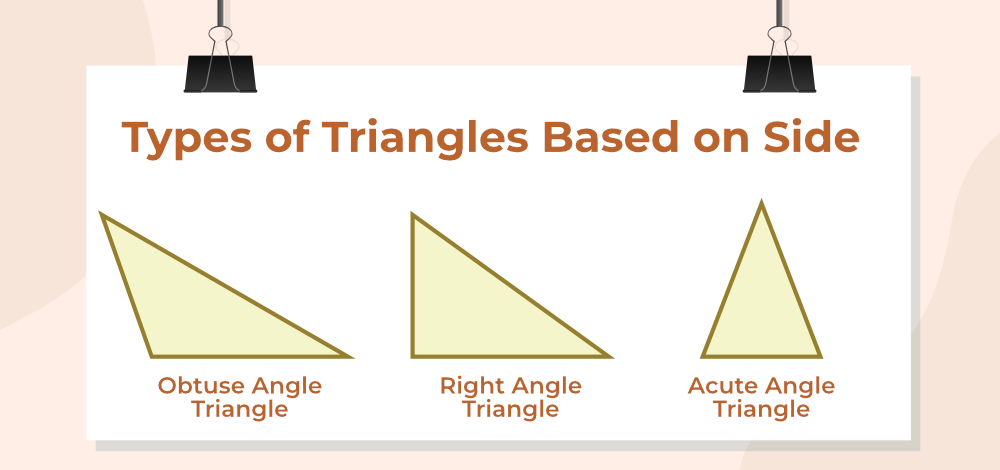### Acute Angled Triangle

In Acute angle triangles, all the angles are greater than 0° and less than 90°. So, it can be said that all 3 angles are acute in nature (angles are lesser than 90°)

Properties of Acute Angled Triangles

• All the interior angles are always less than 90° with different lengths of their sides.
• The line that goes from the base to the opposite vertex is always perpendicular.

### Obtuse Angled Triangle

In an obtuse angle Triangle, one of the 3 sides will always be greater than 90°, and since the sum of all three sides is 180°, the rest of the two sides will be less than 90°(angle sum property).

Properties of Obtuse Angled Triangle

• One of the three angles is always greater than 90°.
• The sum of the remaining two angles is always less than 90° (angle sum property).
• The circumference and the orthocenter of the obtuse angle lie outside the triangle.
• The Incenter and the centroid lie inside the triangle.

### Right Angled Triangle

When one angle of a triangle is exactly 90°, then the triangle is known as the Right Angle Triangle.

Properties of Right-angled Triangle

• A Right-angled Triangle must have one angle exactly equal to 90°, it may be scalene or isosceles but since one angle has to be 90°, hence, it can never be an equilateral triangle.
• The side opposite 90° is called Hypotenuse.
• The sides are adjacent to the 90° are base and perpendicular.
• Pythagoras Theorem: It is a special property for Right-angled triangles. It states that the square of the hypotenuse is equal to the sum of the squares of the base and perpendicular i.e. AC2 = AB2 + BC2

## Solved Examples on Triangles

Example 1: In the triangle. ∠ACD = 120°, and ∠ABC = 60°. Find the type of Triangle.

Solution:

In the above figure, we can say, ∠ACD = ∠ABC + ∠BAC (Exterior angle Property)

120° = 60° + ∠BAC

∠BAC = 60°

∠A + ∠B + ∠C = 180°

∠C OR ∠ACB = 60°

Since all the three angles are 60°, the triangle is an Equilateral Triangle.

Example 2: The triangles with sides of 5 cm, 5 cm, and 6 cm are given. Find the area and perimeter of the Triangle.

Solution:

Given, the sides of a triangle are 5 cm, 5 cm, and 6 cm

Perimeter of the triangle = (5 + 5 + 6) = 16 cm

Semi Perimeter = 16 / 2 = 8 cm

Area of triangle = √s(s – a)(s – b)(s – c) (Using Heron’s Formula)

= √8(8 – 5)(8 – 5)(8 – 6)

= √144 = 12 cm2

Example 3: In the Right-angled triangle, ∠ACB = 60°, and the length of the base is given as 4cm. Find the area of the Triangle.

Solution:

Using trigonometric formula of tan60°,

tan60° = AB / BC = AB  /4

AB = 4√3cm

Area of Triangle ABC = 1/2
= 1/2 × 4 × 4√3
= 8√3 cm2

Example 4: In ΔABC if ∠A+ ∠B = 55°. ∠B + ∠C = 150°, Find angle B separately.

Solution:

Angle Sum Property of a Triangle says ∠A+ ∠B+ ∠C= 180°

Given:
∠A+ ∠B = 55°
∠B+ ∠C = 150°

∠A+ ∠B+ ∠B+ ∠C= 205°
180°+ ∠B= 205°

∠B = 25°

## FAQs on Triangles

### Q1: What is a triangle?

A triangle is a polygon, with three sides and three vertices. A triangle also has three angles and the sum of all three angles of the triangle is 180 degrees.

### Q2: What are the triangle formulas?

The main triangle formulas are,

Area of triangle

A = [(½) b × h]

where ‘b‘ is the base and ‘h‘ is the height of the triangle.

Perimeter of a triangle

P = (a + b + c)

where ‘a‘, ‘b‘, and ‘c‘ are the sides of the triangle.

### Q3: What are the six basic types of triangles?

The six basic types of triangles are,

• Acute angle triangles
• Obtuse angle triangles
• Right angle triangles
• Scalene Triangles
• Isosceles triangles
• Equilateral triangles

### Q4: What are the properties of triangles?

Some of the important properties of triangles are:

• Sum of all three angles of the triangle is equal to 180 degrees.
• Equal angles have equal sides opposite them.
• Sum of the length of any two sides of a triangle is always greater than the third side.

### Q5: What is the difference between scalene, isosceles, and equilateral triangles?

On the basis of sides triangles are of three types:

• Scalene Triangle: A triangle with all three sides unequal is called a Scalene triangle.
• Isosceles Triangle: A triangle in which any two sides are equal is an Isosceles triangle.
• Equilateral Triangle: A triangle with all three sides equal is called an Equilateral triangle.

### Q6: What is the difference between an acute triangle, an obtuse triangle, and a right triangle?

On the basis of angles triangles are of three types:

• Acute Triangle: A triangle with all three angles as acute angles is called an acute angle triangle.
• Obtuse Triangle: A triangle with any one angle as an obtuse angle is called an obtuse angle triangle.
• Right Triangle: A triangle with any one angle as a right angle is called a right angle triangle.

### Q7: What is the triangle where two sides are equal?

A triangle where two sides are equal is called an Isosceles triangle.

### Q8: How many triangles are in a star?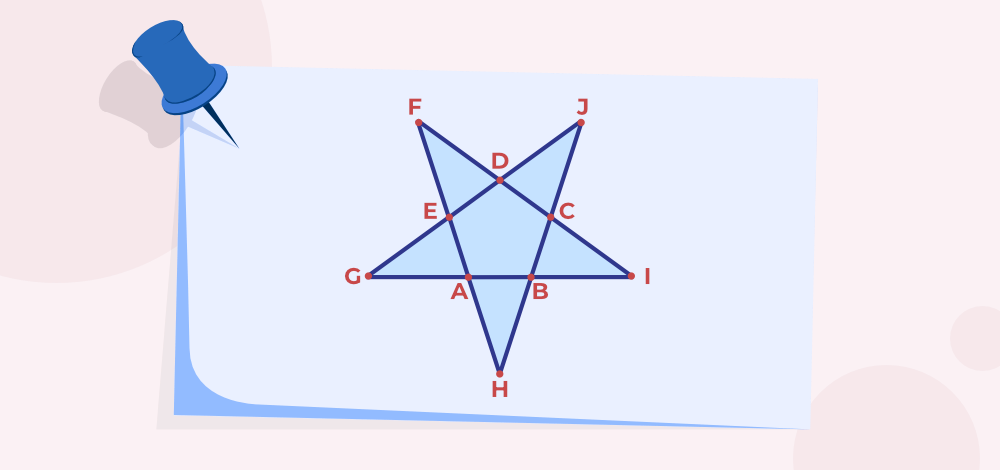A star has 10 triangles that are,

∆ABH, ∆BIC, ∆CJD, ∆DFE, ∆EGA, ∆DGI, ∆GJB, ∆FAI, ∆JGB and ∆EHJ

### Q9: How many triangles can a pentagon be divided into?